# Thermochemistry 2. Re-arrange as appropriate and combine the first two equations to get the third equation...

Thermochemistry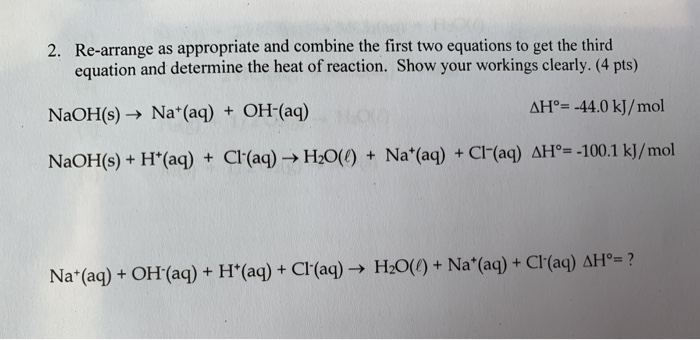2. Re-arrange as appropriate and combine the first two equations to get the third equation and determine the heat of reaction. Show your workings clearly.(4 pts) AH -44.0 kJ/mol NAOH(s) Na(aq) + OH-(aq) NaOH(s)+ H'(aq) + Cl (aq) H2O() + Na (aq) + Cr(aq) AH=-100.1 kJ/mol H2O()+ Na (aq) + Cl(aq) AH°=? Na (aq)+OH (aq) + H*(aq) + Cl'(aq)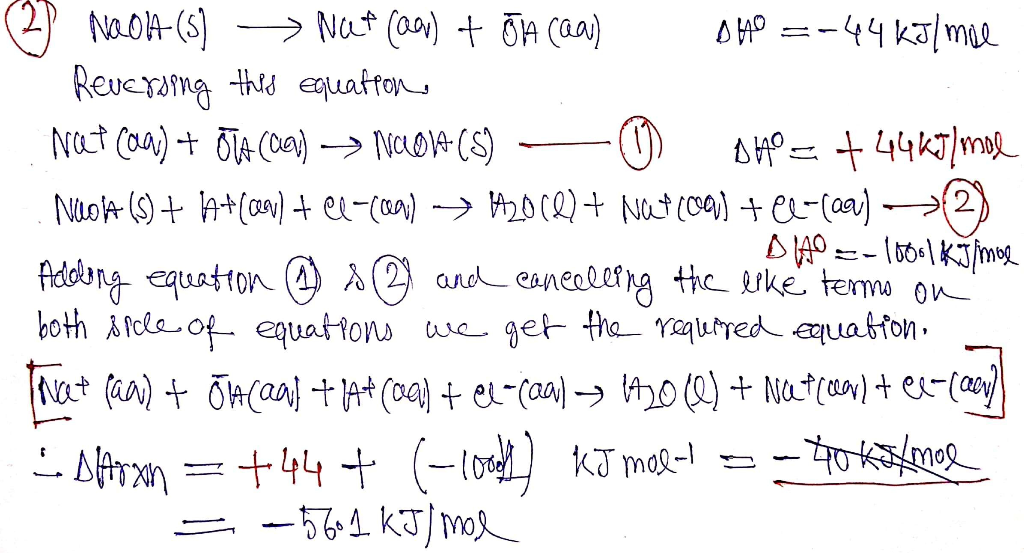#### Earn Coin

Coins can be redeemed for fabulous gifts.

Similar Homework Help Questions
• ### 2. Re-arrange as appropriate and combine the first two equations to get the third equation and...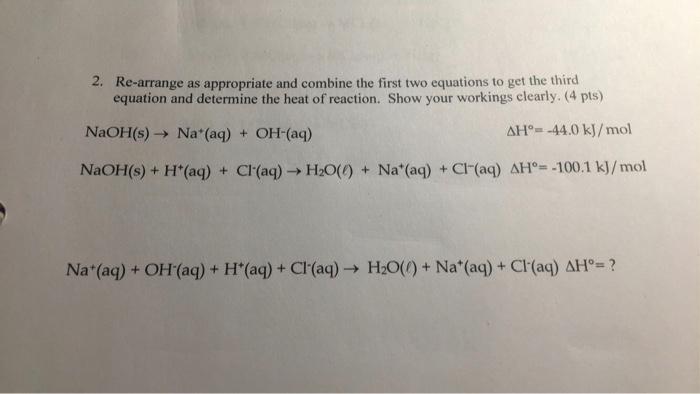2. Re-arrange as appropriate and combine the first two equations to get the third equation and determine the heat of reaction. Show your workings clearly. (4 pts) NaOH(s) Na+ (aq) + OH-(aq) AH°= -44.0 kJ/mol NaOH(s) + H(aq) + Cl(aq) → H2O(0) + Na*(aq) + Cl(aq) AH--100.1 kJ/mol Na*(aq) + OH (aq) + H*(aq) + Cl'(aq) → H2O(0) + Na+ (aq) + Cl(aq) AH°= ?

• ### Combine 2 equations to obtain the third reaction equation

Reaction 1:  Solid sodium hydroxide dissolves in water to form an aqueous solution of ions.NaOH(s) → Na+(aq) + OH–(aq)   ΔH1 = ? Reaction 2:  Solid sodium hydroxide reacts with aqueous hydrochloric acid to form water and an aqueous solution of sodium chloride.NaOH(s) + H+(aq) + Cl–(aq) → H2O(l) + Na+(aq) + Cl–(aq)   ΔH2 = ? Reaction 3:  Solutions of aqueous sodium hydroxide and hydrochloric acid react to form water and aqueous sodium chloride.Na+(aq) + OH–(aq) + H+(aq) + Cl–(aq) → H2O(l) + Na+(aq) +...

• ### Is the enthalpy change just the opposite of the heat absorbed? Do you use that opposite...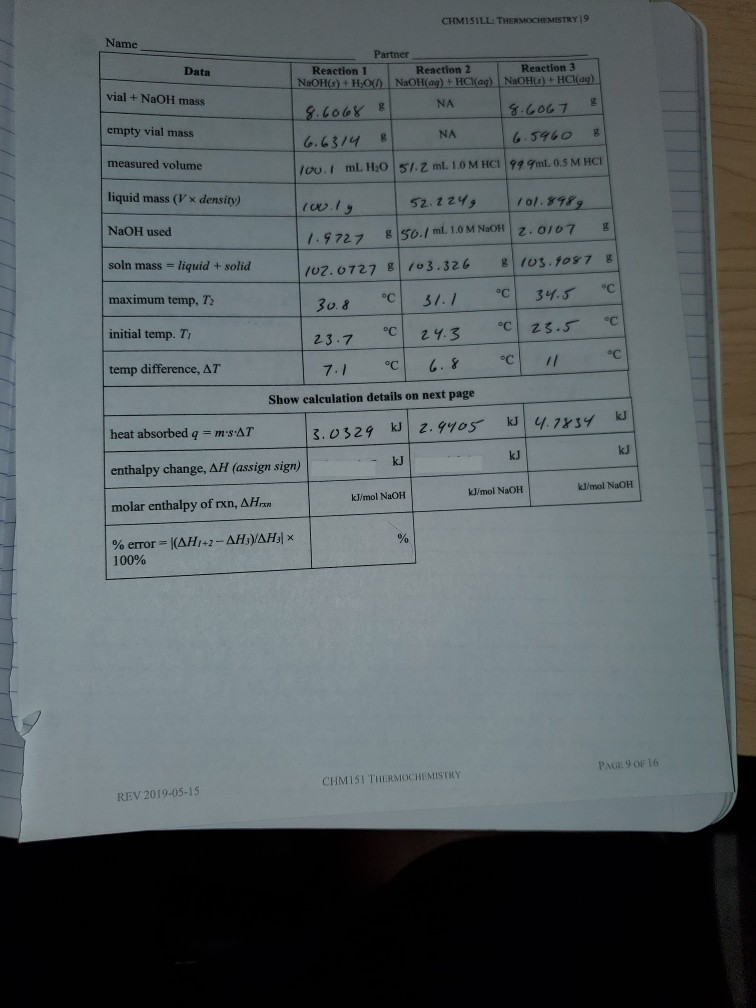Is the enthalpy change just the opposite of the heat absorbed? Do you use that opposite number to calculate the molar enthalpy? CHMISILLE THERMOCHEMISTRY 19 Name Data / vial + NaOH mass / Partner Reaction 1 Reaction 2 Reaction 3 NaOH(s) + H2O( NaOH(aq) + HCl(aq) NaOH(s) + HCl(aq) 8.6068 8 NA 18.6067 B 6.6314 B NA 16.5960 100.1 mL H0 51.Z ml 1.0 M HCI 999mL. 0.5 M HCI / empty vial mass measured volume / liquid mass (V...

• ### NAME INSTRUCTOR DATE SECTION GROUP POST-LABORATORY QUESTIONS THERMOCHEMISTRY: ACID-BASE NEUTRALIZATION 1. Use Hess's Law and the...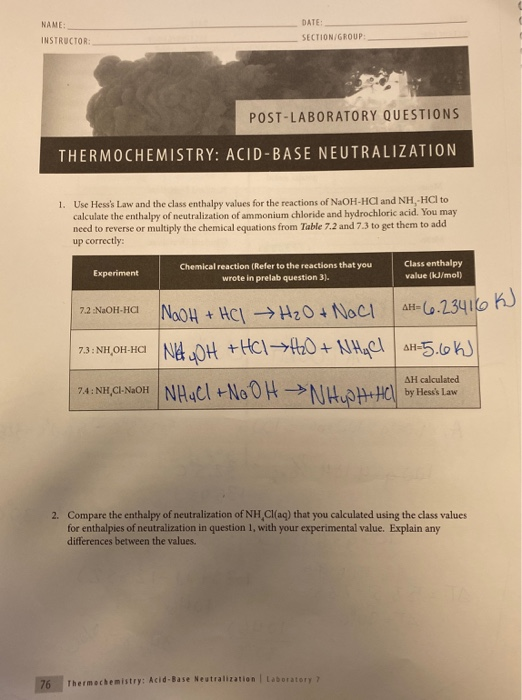NAME INSTRUCTOR DATE SECTION GROUP POST-LABORATORY QUESTIONS THERMOCHEMISTRY: ACID-BASE NEUTRALIZATION 1. Use Hess's Law and the class enthalpy values for the reactions of NaOH-HC and NH, HCl to calculate the enthalpy of neutralization of ammonium chloride and hydrochloric acid. You may need to reverse or multiply the chemical equations from Table 7.2 and 7.3 to get them to add up correctly: Experiment Chemical reaction (Refer to the reactions that you wrote in prelab question 3). Class enthalpy value (kJ/mol 7.2...

• ### How do I approach this? The temperature are in degrees Celcius btw. Thank you! Reaction 1:...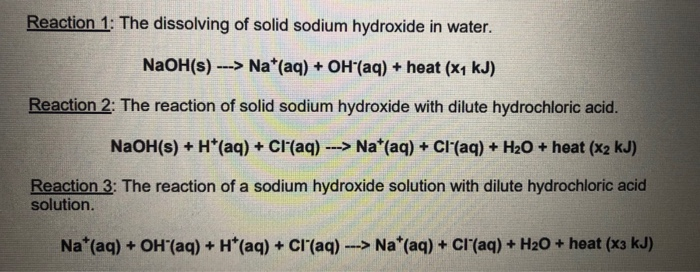How do I approach this? The temperature are in degrees Celcius btw. Thank you! Reaction 1: The dissolving of solid sodium hydroxide in water. NaOH(s) ---> Nat(aq) + OH"(aq) + heat (x1 kJ) Reaction 2: The reaction of solid sodium hydroxide with dilute hydrochloric acid. NaOH(s) + H+ (aq) + Cl'(aq) ---> Nat(aq) + Cl(aq) + H2O + heat (x2 kJ) Reaction 3: The reaction of a sodium hydroxide solution with dilute hydrochloric acid solution. Na*(aq) + OH"(aq) + H+...

• ### Equations AS-re/T, AS system= ES® (products) - ES (reactants); AS univ=AS sys+AS sur; AGⓇ-AH- AGG products...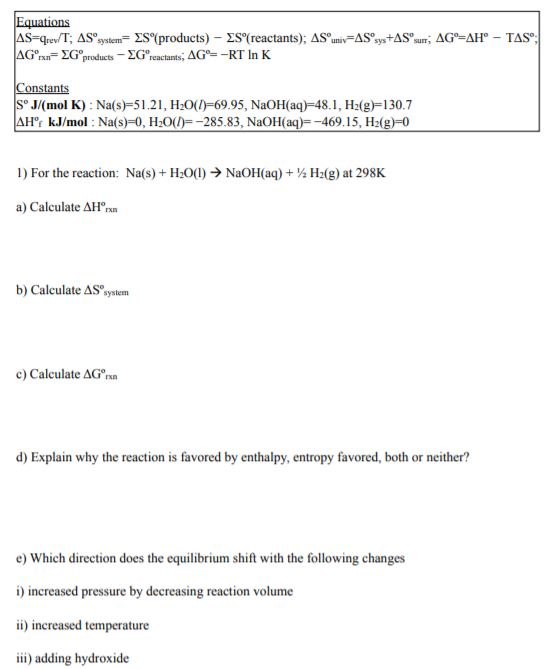Equations AS-re/T, AS system= ES® (products) - ES (reactants); AS univ=AS sys+AS sur; AGⓇ-AH- AGG products - Gractants; AGⓇ--RT In K TAS: Constants SJ/mol K): Na(s)=51.21, H20(1=69.95, NaOH(aq)-48.1, H2(g)=130.7 AHºr kJ/mol : Na(s)=0, H2O(I=-285.83, NaOH(aq)=-469.15, H2(g)=0 1) For the reaction: Na(s) + H2O(l) → NaOH(aq) + H2(g) at 298K a) Calculate AHºrn b) Calculate AS system c) Calculate AG® d) Explain why the reaction is favored by enthalpy, entropy favored, both or neither? e) Which direction does the equilibrium shift...

• ### Calculations Sheet Thermochemistry 2 Part 2: Hydrochloric Acid + Magnesium The strategy for these calculations are...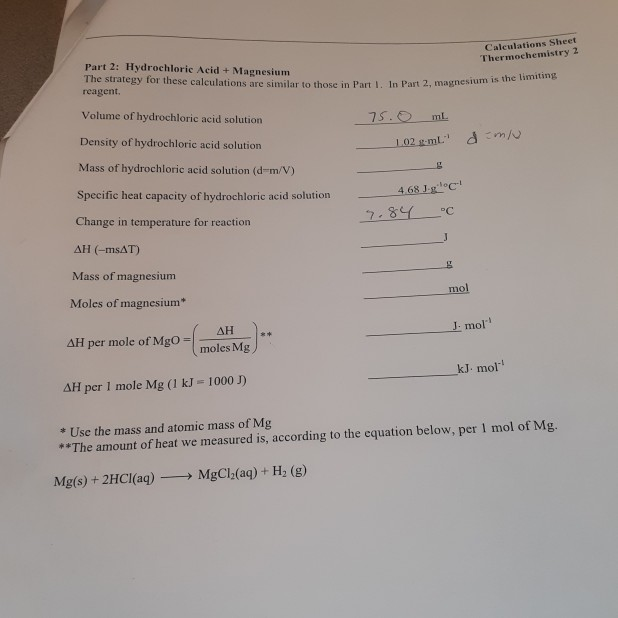Calculations Sheet Thermochemistry 2 Part 2: Hydrochloric Acid + Magnesium The strategy for these calculations are similar to those in Part I. In Part 2, magnes reagent. Volume of hydrochloric acid solution 75.0 mL Part 1. In Part 2, magnesium is the limiting Density of hydrochloric acid solution 102 g ml." dem Mass of hydrochloric acid solution (d-m/V) Specific heat capacity of hydrochloric acid solution 4.68 9.84 °C C Change in temperature for reaction AH(-MSAT) Mass of magnesium mol Moles...

• ### D Question 5 2 pts Suppose 160 mL of 8.0 M NaOH(aq) is added to 167...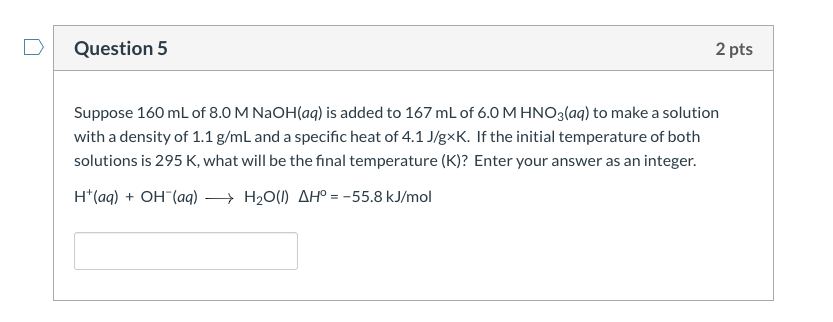D Question 5 2 pts Suppose 160 mL of 8.0 M NaOH(aq) is added to 167 mL of 6.0 M HNO3(aq) to make a solution with a density of 1.1 g/mL and a specific heat of 4.1 J/gxK. If the initial temperature of both solutions is 295 K, what will be the final temperature (K)? Enter your answer as an integer. H(aq) + OH(aq) + H2O(1) AH° = -55.8 kJ/mol

• ### Pre-lab Questions for Experiment #11 Hess's Law with Computers 1) Using the following data, NaOH ()...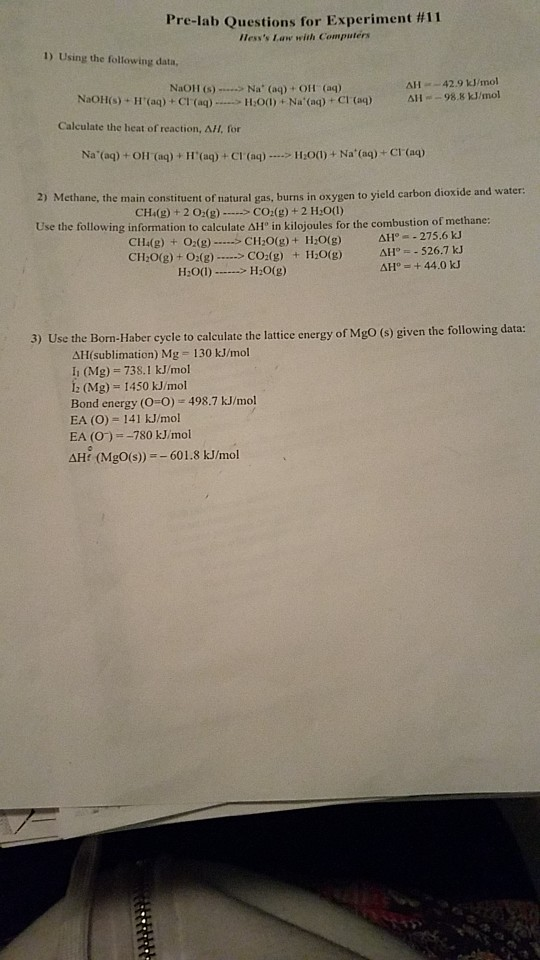Pre-lab Questions for Experiment #11 Hess's Law with Computers 1) Using the following data, NaOH () - Na' (aq) + OH (aq) ΔΙΙ "-42.9 kJ/mol =-98.8 kJ/mol NaOHis).HUaq) + Cl.(aq)....-11:0(1) + Na. (ap-Craq) Calculate the heat of reaction, AH, for ΔΙΙ 2) Methane, the main constituent of natural gas, burns in oxygen to yield carbon dioxide and water: CH(g) + 2 02(g)CO(g) +2 H:00) Use the following information to calculate ΔΗ, in kilojoules for the combustion of methane CH(g) +...

• ### I reuploaded clearer pictures 1 Write net ionic equations for a Reaction 1 All- b. Reaction...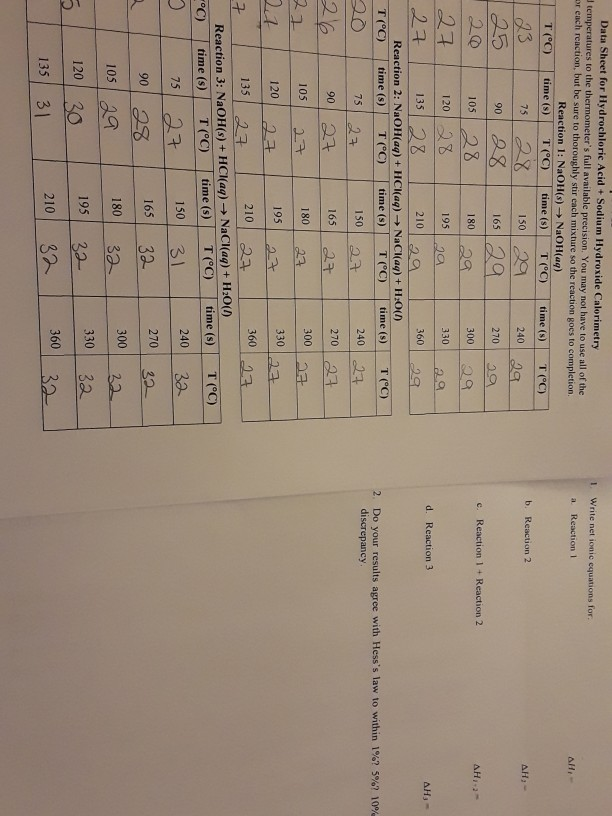I reuploaded clearer pictures 1 Write net ionic equations for a Reaction 1 All- b. Reaction 2 AH:- c. Reaction 1 + Reaction 2 AH- d. Reaction 3 AH- 2. Do your results agree with Hess's law to within 1% discrepancy 5%? 10% Data Sheet for Hydrochloric Acid + Sodium Hydroxide Calorimetry temperatures to the thermometer's full available precision. You may not have to use all of the er each reaction, but be sure to thoroughly stir cach mixture so...